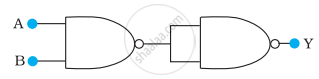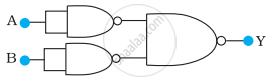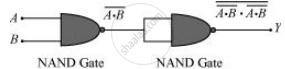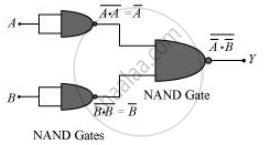# You are given two circuits as shown in following figure, which consist of NAND gates. Identify the logic operation carried out by the two circuits. - Physics

Numerical

You are given two circuits as shown in following figure, which consist of NAND gates. Identify the logic operation carried out by the two circuits.

(a)(b)#### Solution

In both the given circuits, A and B are the inputs and Y is the output.

a) The output of the left NAND gate will be bar("A"."B"), as shown in the following figure.Hence, the output of the combination of the two NAND gates is given as:

"Y" = bar((bar("A.B")).(bar("A.B")))= bar(bar("AB")) + bar(bar("AB")) = "AB"

Hence, this circuit functions as an AND gate.

(b) bar"A" is the output of the upper left of the NAND gate and bar"B" is the output of the lower half of the NAND gate, as shown in the following figure.Hence, the output of the combination of the NAND gates will be given as:

"Y"  = bar"A".bar"B" = bar(bar"A") + bar(bar"B") = "A"+"B"

Hence, this circuit functions as an OR gate.

Concept: Digital Electronics and Logic Gates
Is there an error in this question or solution?

#### APPEARS IN

NCERT Physics Part 1 and 2 Class 12
Chapter 14 Semiconductor Electronics: Materials, Devices and Simple Circuits
Exercise | Q 14.13 | Page 499
NCERT Class 12 Physics Textbook
Chapter 14 Semiconductor Electronics: Materials, Devices and Simple Circuits
Exercise | Q 17 | Page 511

Share